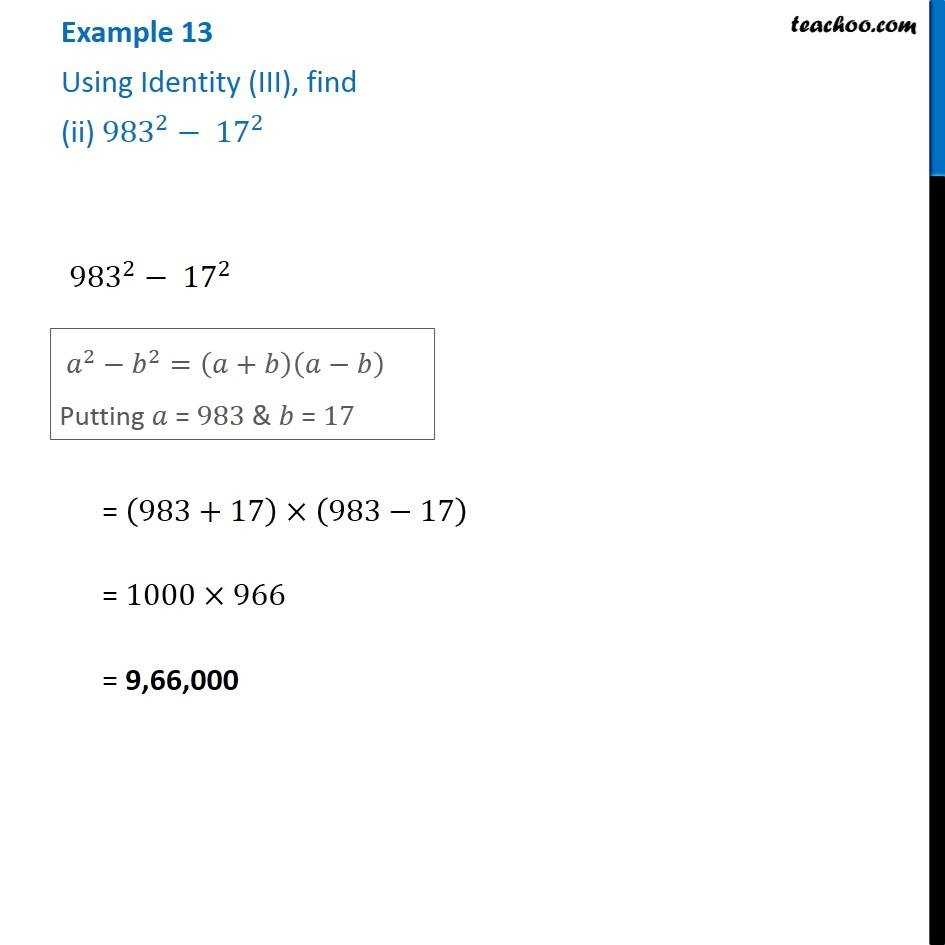Examples

Chapter 8 Class 8 Algebraic Expressions and Identities
Serial order wiseLearn in your speed, with individual attention - Teachoo Maths 1-on-1 Class

### Transcript

Example 13 Using Identity (III), find (ii) 〖983〗^2− 〖17〗^2 〖983〗^2− 〖17〗^2 𝑎^2−𝑏^2=(𝑎+𝑏)(𝑎−𝑏) Putting 𝑎 = 983 & 𝑏 = 17 = (983+17)×(983−17) = 1000×966 = 9,66,000